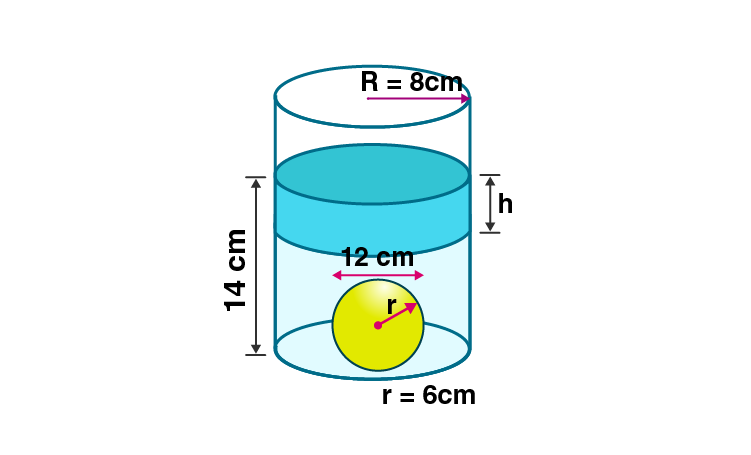Newbie

# There is water to a height of 14 cm in a cylindrical glass jar of radius 8 cm. Inside the water there is a sphere of diameter 12 cm completely immersed. By what height will the water go down when the sphere is removed?

• 0

ICSE Board Question Based on Mensuration Chapter of M.L Aggarwal for class10
The height and radius of a cylindrical glass jar is given which is having water in it.
Inside the water there is a sphere of given diameter completely immersed. By what height will the water go down when the sphere is removed?
This is the Question Number 16, Exercise 17.5 of M.L Aggarwal.

Share

1.Given radius of the glass jar, R = 8 cm

Diameter of the sphere = 12 cm

Radius of the sphere, r = 12/2 = 6 cm

When the sphere is removed from the jar, volume of water decreases.

Let h be the height by which water level will decrease.

Volume of water decreased = Volume of the sphere

R2h = (4/3)r3

82h = (4/3)63

h = (4/3)×6×6×6/(8×8)

= 18/4 = 9/2 = 4.5 cm

Hence the height by which water level decreased is 4.5 cm.

• 0